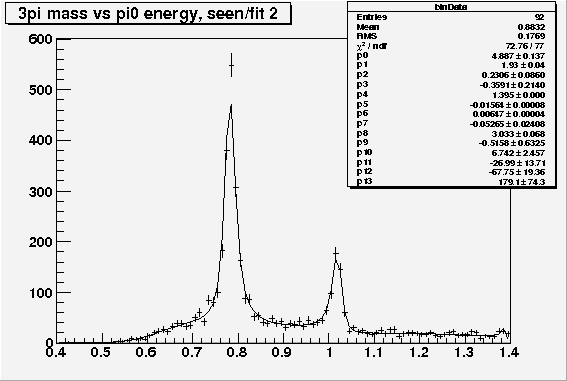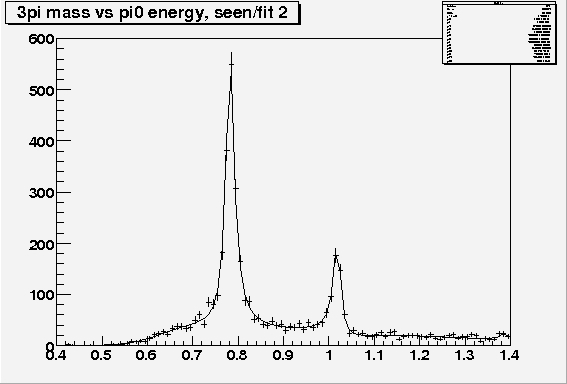# Can someone PLEASE check this!

Hi there,

I’m having serious problems with a fit. Basically I fit a function to a histogram using TH1->Fit(). The fit works but then I save the fitted function to a TFile using

``````        TFile * om = new TFile("Omega.root","UPDATE");
myfit->Write("fit",TObject::kOverwrite);
om.Close();``````

When I open the fit again it has changed. The parameters appear to be ‘re-set’ somehow.
Any suggestions?
The whole thing is behaving strangly. This program used to work and the only thing I’ve changed is the fitted function. It is a very complicated function using TComplex.
I’d really like someone to make sure I’m using TComplex in the correct way, so I’ve included it below. It takes at least 20 minutes to fit, which seems like a long time, but don’t know if this is reasonable for a function with 14 parameters?

The function has been written as a class to make it quicker. The parts that only need doing once are done in Load(), then the parts that need to be done for every iteration of the fit are in BukinFn().

So could you please see if this function is written in the right way, or if there are any ways the fitting time could be reduced:

``````///////////////////////////////////////////////////////////////////////////////////////////////////////////////
///////Bukin Class: BukinFn
///////
///////This is Bukin's complete function for the shape of  the omega and
////// phi peak.
///////
///////It has 8 parameters: N, mu, c, d, Mx, Gammax, sigma and phi.
///////It also has a background function with another 5 parameters.
///////
//////////////////////////////////////////////////////////////////////////////////////////////////////////////

#include "TMath.h"
#include "TComplex.h"

//Define class Bukin
class Bukin {

//Constructor
Double_t oMASS;
Double_t pMASS;
Double_t oWIDTH;
Double_t pWIDTH;

Double_t rho1;
Double_t rho2;
Double_t rho;

Double_t bukin;
Double_t bg;

Double_t xMASS;
Double_t xWIDTH;

Double_t N;
Double_t mu;
Double_t c;
Double_t d;
Double_t sigma;
Double_t phi;

Double_t b0;
Double_t b1;
Double_t b2;
Double_t b3;
Double_t b4;
Double_t b5;

Double_t rhosinsq;
Double_t aRe;
Double_t bRe;

Double_t x1;
Double_t x2;
Double_t x3;
Double_t x4;
Double_t x5;

//I don't know why but it crashes if 'all' is defined here.
//TComplex all;
TComplex a;
TComplex b;
TComplex po;
TComplex px;
TComplex op;
TComplex ox;
TComplex xp;
TComplex xo;
TComplex ptemp;
TComplex otemp;
TComplex xtemp;
TComplex cd;
TComplex cminusd;
TComplex i;
TComplex sqbracket1;
TComplex sqbracket2;
TComplex sqbracket3;
cout << "constructor done" << endl;

public:

//Function Load(): Does anything that doesn't depend on the parameters.
//Load() is performed once before the fitting begins.

oMASS = 0.782;
pMASS = 1.020;
oWIDTH = 0.008*0.5;
pWIDTH = 0.004*0.5;

rho1 = (4.0*TMath::Power(pMASS-oMASS,2.0))+TMath::Power(pWIDTH+oWIDTH,2.0);
rho2 = (2.0*(pWIDTH+oWIDTH)*TMath::Sqrt(pWIDTH*oWIDTH));
rho = (rho1+rho2)/(rho1-rho2);

bukin = 0.0;
all = TComplex();
bg = 0.0;

}

//Function BukinFn(): returns the y-value corresponding to a given x.
//The function describes the shape of the omega and phi peaks.
Double_t BukinFn(Double_t *x, Double_t *par) {

xMASS = par;
xWIDTH = par*0.5;

N = par;
mu = par;
c = par;
d = par;
sigma = par;
phi = par;

b0 = par;
b1 = par;
b2 = par;
b3 = par;
b4 = par;
b5 = par;

rhosinsq = rho*TMath::Sin(mu)*TMath::Sin(mu);

aRe = TMath::Sqrt(pWIDTH/8.0*TMath::Pi())*(TMath::Sqrt(1.0+rhosinsq)+TMath::Cos(mu));
bRe = TMath::Sqrt(oWIDTH/8.0*TMath::Pi())*(TMath::Sqrt(1.0+rhosinsq)-TMath::Cos(mu));

b = TComplex(bRe, 0);
a = TComplex(aRe*TMath::Cos(phi), aRe*TMath::Sin(phi));

po = TComplex(pMASS-oMASS, pWIDTH+oWIDTH);
px = TComplex(pMASS-xMASS, pWIDTH+xWIDTH);
op = TComplex(oMASS-pMASS, oWIDTH+pWIDTH);
ox = TComplex(oMASS-xMASS, oWIDTH+xWIDTH);
xp = TComplex(xMASS-pMASS, xWIDTH+pWIDTH);
xo = TComplex(xMASS-oMASS, xWIDTH+oWIDTH);

ptemp = TComplex((1.17741*sigma)+pWIDTH, x-pMASS);
otemp = TComplex((1.17741*sigma)+oWIDTH, x-oMASS);
xtemp = TComplex((1.17741*sigma)+xWIDTH, x-xMASS);

cd = TComplex(c, d);
cminusd = TComplex(c, d*(-1));

i = TComplex(0, 1);

sqbracket1 = TComplex();
sqbracket2 = TComplex();
sqbracket3 = TComplex();

sqbracket1 = (a/(2*pWIDTH)) - ((i*b)/po) + ((i*cd)/px);
sqbracket2 = ((i*a)/op) - (b/(2*oWIDTH)) + ((i*cd)/ox);
sqbracket3 = ((i*a)/xp) - ((i*b)/xo) + (cd/(2*xWIDTH));

all = ((a/ptemp)*sqbracket1) - ((b/otemp)*sqbracket2) + ((cminusd/xtemp)/sqbracket3);

bukin = N*2*all.Re();

//Background function: 6 parameters; b0, b1, b2, b3, b4, b5.

x1 = x-1;
x2 = x1*x1;
x3 = x2*x1;
x4 = x3*x1;
x5 = x4*x1;

bg = TMath::Exp(b0 + (b1*x1) + (b2*x2) + (b3*x3) + (b4*x4) + (b5*x5));

return bukin + bg;

}
};``````

Any help would be really appreciated, I’ve been trying to make this work for ages and I have a deadline soon!
Thank you,
Kim

Hi,

the code for the function looks to me having several compilation error.
Are you running the code in compiled mode ?
If you want it to run faster, you should compile it.

I notice you have your fitted function defined as a member function. Presently you cannot use in TH1:Fit in ROOT a non static member function of a class.

If you need help, please send the shortest program running your fit.

Regards,
Lorenzo

Here’s the code for the program which runs BukinFn.C:

``````////////////////////////////////////////////////////////////////
////// Fitting Function  (for BukinFn)
//////
////// Written by Kim Alwyn   (30/03/2006)
////// Editted by Kim Alwyn   (21/05/2006)
//////                        (27/09/2006)
//////
//////
////// This takes the mass spectrums from the data.
////// Then BukinFn is fitted to the spectrum.
////// The fit is saved onto a file called newOmega.root.
//////
////////////////////////////////////////////////////////////////

#include "BukinFn.C"
#include "TMath.h"
#include <sstream>
#include <string>

#include <cstdlib>
#include <iostream>
#include <fstream>
#include <stdlib>
#include [itex]

#include <TROOT>
#include <TApplication>

#include <TFile>
#include <TString>
#include <TChain>
#include <TCanvas>
#include <TH1>
#include <TH2>
#include <TH2F>
#include <TF1>
#include <TStyle>
#include <TPostScript>
#include <TBox>
#include <TGraph>
#include <TGraphErrors>
#include <TLine>
#include <TText>
#include <TSystem>
#include <TRandom3>
#include <THtml>
#include <TVector3>
#include <TVector>

using namespace std;

//define a class Bukin called "buk".
class Bukin buk;

//define a function MyFn.
//this simply returns the BukinFn so that it is in the right form to be used
//in the definition of TF1 "myfit".
Double_t MyFn(Double_t *x, Double_t *params){
return buk.BukinFn(x, params);
}

int Fitting()
{

TFile * dat = new TFile("data_15-08-06.root");

TH2F * h1;
h1 = (TH2F*)dat->Get("h2d710");

c1->Clear();

//Function Load() performs actions that only need to be done once.

//Define myfit.
TF1 * myfit = new TF1("myfit", MyFn, 0.4, 1.4, 14);

myfit->SetParameters(6.2, 2.06, 0.846, 0.064, 1.53, 0.136, 0.0155, 0.0327, 2.683, 4.16, -9.4);
myfit->SetParameter(11, 16.8);       //->SetParameters only takes 10 parameters
myfit->SetParameter(12, 0.0);
myfit->SetParameter(13, 0.0);

//myfit->SetParameters(4.885, 1.935, 0.2238, -0.3353, 1.395, -0.01562, 0.006453, -0.05269, 3.033, -0.5169, 6.753);
//myfit->SetParameter(11, -27.12);
//myfit->SetParameter(12, -68.);
//myfit->SetParameter(13, 179.3);

myfit->SetLineWidth(0.1);

//Get projection of data.
TH1 * h2 = h1->ProjectionX("binData", 1, 1, "e");
h2->Draw();

cout << "Fitting bin 1..." <<endl>Fit("myfit", "R0", "", 0.4, 1.4);
cout << "Fit done " <<endl>Draw("same");

gStyle->SetOptStat();
gStyle->SetOptFit();

c1->SaveAs("screen1.eps"); //This output gives a "goodScreen.eps" which is attached

TFile * om = new TFile("newOmega1.root","UPDATE");

h2->Write("SeenData",TObject::kOverwrite);
myfit->Write("fitSD",TObject::kOverwrite);    //Once the output is saved it gives "badScreen.eps" which is attached
om->Close();

return 1;

}``````

Ive also attached the input roof file: “data_15-08-06.root”
A plot of the fit before it’s saved: “goodScreen.jpg”
A plot of the fit after it’s saved: “badScreen.jpg”

So I just need to know why it changes when it’s saved.data_15-08-06.root (121 KB)

Your program contained still some errors. Here attached is the fixed version.

Since you are not using a formula for the function, but you pass your defined one, you cannot store the function evaluation in a file.
What is stored are the function points. You hae this effect because only the default 100 points are stored. For better precision call
TF1::SetNpx(npx) with npx larger, like 10000.

The fit is fast in compiled mode, so I guess you were running in interpreted mode.

Regards, Lorenzo
BukinFn.C (4.69 KB)
FittingComplex.C (3.44 KB)